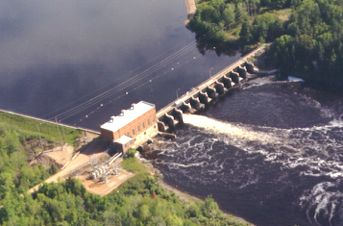# How Hydropower WorksHydropower plants capture the energy of falling water to generate electricity. A turbine converts the kinetic energy of falling water into mechanical energy. Then a generator converts the mechanical energy from the turbine into electrical energy.

Hydroplants range in size from "micro-hydros" that power only a few homes to giant dams like Hoover Dam that provide electricity for millions of people.

The photo on the right shows the Alexander Hydroelectric Plant on the Wisconsin River, a medium-sized plant that produces enough electricity to serve about 8,000 people.

#### Parts of a Hydroelectric Plant

Most conventional hydroelectric plants include four major components (see graphic below):

1. Dam. Raises the water level of the river to create falling water. Also controls the flow of water. The reservoir that is formed is, in effect, stored energy.
2. Turbine. The force of falling water pushing against the turbine's blades causes the turbine to spin. A water turbine is much like a windmill, except the energy is provided by falling water instead of wind. The turbine converts the kinetic energy of falling water into mechanical energy.
3. Generator. Connected to the turbine by shafts and possibly gears so when the turbine spins it causes the generator to spin also. Converts the mechanical energy from the turbine into electric energy. Generators in hydropower plants work just like the generators in other types of power plants.
4. Transmission lines. Conduct electricity from the hydropower plant to homes and business.#### How Much Electricity Can a Hydroelectric Plant Make?

The amount of electricity a hydropower plant produces depends on two factors:

1. How Far the Water Falls. The farther the water falls, the more power it has. Generally, the distance that the water falls depends on the size of the dam. The higher the dam, the farther the water falls and the more power it has. Scientists would say that the power of falling water is "directly proportional" to the distance it falls. In other words, water falling twice as far has twice as much energy.
2. Amount of Water Falling. More water falling through the turbine will produce more power. The amount of water available depends on the amount of water flowing down the river. Bigger rivers have more flowing water and can produce more energy. Power is also "directly proportional" to river flow. A river with twice the amount of flowing water as another river can produce twice as much energy.

#### Can I Figure Out How Much Energy a Dam in My Area Can Make?

Sure. It's not that hard.

Let's say that there is a small dam in your area that is not used to produce electricity. Maybe the dam is used to provide water to irrigate farmlands or maybe it was built to make a lake for recreation. As we explained above, you need to know two things:

1. How far the water falls. From talking to the person who operates the dam, we learn that the dam is 10 feet high, so the water falls 10 feet.
2. Amount of water flowing in the river. We contact the United States Geological Survey, the agency in the U.S. that measures river flow, and learn that the average amount of water flowing in our river is 500 cubic feet per second.

Now all we need to do is a little mathematics. Engineers have found that we can calculate the power of a dam using the following formula:

Power = (Height of Dam) x (River Flow) x (Efficiency) / 11.8

 Power The electric power in kilowatts (one kilowatt equals 1,000 watts). Height of Dam The distance the water falls measured in feet. River Flow The amount of water flowing in the river measured in cubic feet per second. Efficiency How well the turbine and generator convert the power of falling water into electric power. For older, poorly maintained hydroplants this might be 60% (0.60) while for newer, well operated plants this might be as high as 90% (0.90). 11.8 Converts units of feet and seconds into kilowatts.

For the dam in our area, lets say we buy a turbine and generator with an efficiency of 80%.

Then the power for our dam will be:

Power = (10 feet) x (500 cubic feet per second) x (0.80) / 11.8 = 339 kilowatts

To get an idea what 339 kilowatts means, let's see how much electric energy we can make in a year.

Since electric energy is normally measured in kilowatt-hours, we multiply the power from our dam by the number of hours in a year.

Electric Energy = (339 kilowatts) x (24 hours per day) x (365 days per year) = 2,969,000 kilowatt hours.

The average annual residential energy use in the U.S. is about 3,000 kilowatt-hours for each person. So we can figure out how many people our dam could serve by dividing the annual energy production by 3,000.

People Served = 2,969,000 kilowatts-hours / 3,000 kilowatt-hours per person) = 990 people.

So our local irrigation or recreation dam could provide enough renewable energy to meet the residential needs of 990 people if we added a turbine and generator.

Note: Before you decide to add hydropower to a dam, have a hydropower engineer review your calculations and consult with the local resource agencies to be sure you can obtain any permits that are required.